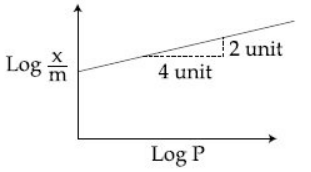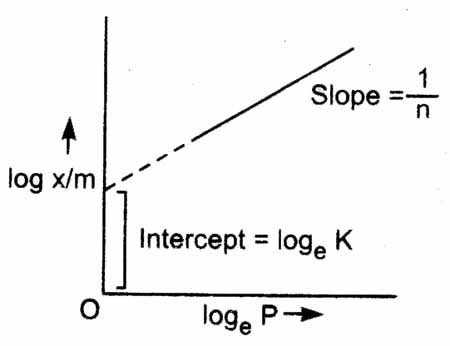Q

# How to solve this problem- - A dsorption of gas follows Freundlich adsorbtin isotherm. In the given plox ,x is the mass of the ga - Surface Chemistry - JEE Main

A dsorption of gas follows Freundlich adsorbtin isotherm. In the given plox ,x is the mass of the gas  adsorbent at pressure P.     is propotional to:• Option 1)

• Option 2)

• Option 3)

• Option 4)

Views

Freundlich gave an empirical relationship between the quantity of gas adsorbed by a unit mass of solid adsorbent and pressure at a particular temperature

- wherein

(n>1)

As we know that:

From the graph

Logarithmic graph of Freundlich isotherm -- wherein

The slope of the straight line gives the value of 1/n. The intercept on y-axis gives the value of log K.

Option 1)

Option 2)

Option 3)

Option 4)

Exams
Articles
Questions